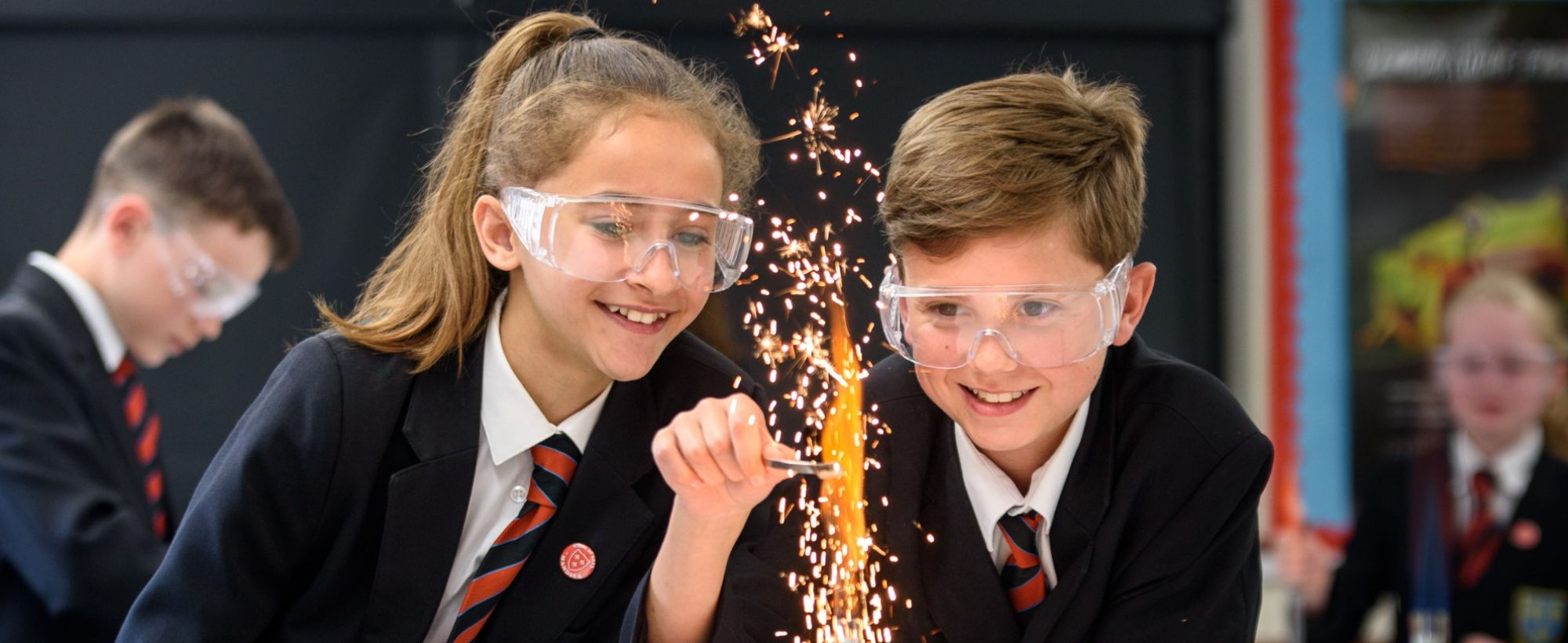•••••# GCSE

## Foundation & Higher Course

Click the logo or link below if you would like to view the full course specification from the Exam Board website.

 Exam Board Qualification SpecificationGCSE Mathematics 9 to 1 1MA1 GCSE Specification (9 – 1) Higher Grades 9, 8, 7, 6, 5, 4 Foundation Grades 5, 4, 3, 2, 1 Paper 1 (33.3%) Non-Calculator Paper 2 (33.3%) Calculator Paper 3 (33.3%) Calculator

The table below is a breakdown of the units studied at GCSE and the format of the assessments.

### Year 11

Units
In Key Stage 4, students develop and apply more sophisticated methods in Number, Geometry, Algebra and Data Handling.
Assessments
These take place towards the end of each half term. Marks are recorded on Go4Schools.
Units
In Key Stage 4, students develop and apply more sophisticated methods in Number, Geometry, Algebra and Data Handling.
Assessment
Students take a full GCSE mock exam at the end of each term in year 11.

Autumn

Statistics
Sampling data
Statistical graphs
Cumulative frequency
Statistics
Classroom based assessment (1 hour)
Transformations
Transformations
Similarity
Congruence and proof
Loci and inequalities

Proportional Reasoning
Fractions and decimals
Ratio and Percentage
Direct and inverse proportion
Proportional Reasoning
Classroom based assessment (1 hour)
Number
Calculation
Number structure
Laws of indices
Standard form
Surds
GCSE Mock Exam 1
Formal Assessment in exam halls.

Spring

Probability
Multiple events
Mutual exclusivity
Venn diagrams
Relative frequency
Tree diagrams
Probability
Classroom based assessment (1 hour)
Brackets and factorising
Iteration

Linear Algebra
Algebraic manipulation
Equations and formulae
Inequalities
Simultaneous equations
Graphs
Linear Algebra
Classroom based assessment (1 hour)
Trigonometry
Pythagoras Theorem
Trigonometry
Sine and cosine rules
Trig graphs
GCSE Mock Exam 2
Formal Assessment in exam halls.

Summer

Geometry
Properties of shapes
Circle theorems
Geometrical proof
Geometry
Classroom based assessment (1 hour)
Further Algebra
Algebraic fractions
Circle equations
Functions
Transformation of graphs

Measure
Surface area & volume
Circles, arcs and sectors
Cylinders, pyramids and spheres.
End of Year Examination
Formal Assessment in exam halls.

GCSE Examination
Formal Assessment in exam halls.